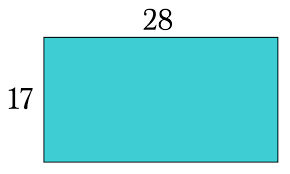# Java Program To Find Perimeter Of Rectangle | 3 Ways

Java program to find the perimeter of a rectangle – In this distinct article, we will discuss the multiple methods used to calculate the perimeter of a rectangle in Java programming.

Suitable examples and sample outputs are included in this piece as well for better interpretation. The compiler has also been added so that you can execute it yourself pretty easily.

The ways to find out the perimeter of a rectangle as per the blog are as follows:

• Using Scanner Class
• Using Command Line Arguments
• Using Static Function

As we all know, a rectangle is a two-dimensional quadrilateral figure in the field of geometry. The opposite sides of a rectangle are equal and parallel in nature.

All of the angles in a rectangle are 90 degrees.As you can see, this is a rectangle with a length of 28 units and a breadth of 17 units.

Thus, the perimeter of a rectangle can be calculated using this formula:

P = 2(l + b)

So, the perimeter of the given rectangle is as follows:

P = 2(28 + 17) = 90 units.

Hence, the methods used to calculate the perimeter of a rectangle in Java programming are as follows:

Output:

Output:

Output:
x

## Java Program to Add Two Matrices – 4 Ways | Programs

Java program to add two matrices – The following Java Code will let you know ...